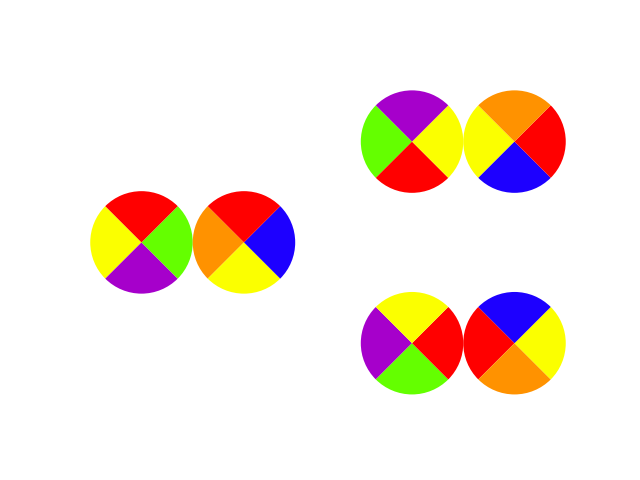### E - Rotate circles

##### Languages: C, C++, Java, Python, ... (details)

There is a board of circles with n rows and m columns. Each circle is divided in four equal regions. Each of the four regions is colored with distinct colors. You can select a circle and rotate it 90 degrees clockwise. A state of the board is beautiful if the two adjacent regions of every pair of neighboring circles have the same color.

Given the initial state of the board, how many different beautiful states you can reach by performing the action as many times as you want. Two states are considered different if there is at least one circle that has different rotations in both states.Image for second test case. In the left of the image is the state of the circles as described in the input. In the right are the two beautiful states. Note that colors are [r]ed, [b]lue, [p]urple, [y]ellow and [o]range.

#### Input

First line contains two integers $n$, $m$ $(1 \le n, m \le 10)$ number of rows and columns of the board respectively. Next $n$ lines contains description of each row of the board. Each line contains $4 \cdot m$ characters, every group of $4$ characters is the description of a circle. Each character $c_i$ is lowercase English letter and represents a color of one of the four regions of the circle. Regions are described in clockwise order starting from upper region. See image for more details.

#### Output

Print one integer, the number of different beautiful states.

#### Sample test(s)

Input
2 2 r b k g r b k g r b k g k g r b
Output
4
Input
1 2 r g p y r b y o
Output
2